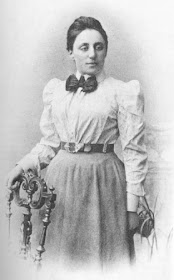### Noether's theoremThe Noether's theorem, discovered by German mathematician Emmy Noether, is one of the most sophisticated theorems in physics, a way to see how group theory, a branch of mathematics believed by many to be abstract, can provide the basis for an important physical concept. The premises of the theory of groups, coupled with the calculus of variations, lead to the conclusions of the theorem: the existence, under certain conditions, of conserved quantities within physical systems.
First of all we start with symmetry, one of the most important concepts for physics, and also the subject og group theory studies. To realize, therefore, this close link, it is enough to have in mind the statement of the theorem:
If a physical system exhibits some continuous symmetry, then there are corresponding observables whose values are constant over time.
A more sophisticated formulation of the theorem, on the other hand, goes something like this:
To every differentiable symmetry generated by local actions there corresponds a conserved current.
This more technical statement links the theorem and the symmetries with some of the most important groups for physics, the Lie groups. In the abstract of the Noether's paper, Invariant Variationsprobleme, in fact, we can read:
The problems in variation here concerned are such as to admit a continuous group (in Lie's sense); the conclusions that emerge for the corresponding differential equations find their more general expression in the theorems formulated in Section I and proved in the following sections.
The Noether's theorem, therefore, ensures that, when a physical system is invariant under the action of the transformations belonging to a Lie group, that is a group in which we are able to differentiate functions, then it certainly exists at least one conserved quantity, and this quantity and its invariance are expressed in the following equation: $\frac{\text{d}}{\text{d} t} \left ( \frac{\partial L}{\partial \dot x_k} \right ) = \frac{\text{d} p_k}{\text{d} t}$
Emmy Noether (1918). Invariante Variationsprobleme. de.wikisource.
English translation by Mort Tavel on arXiv

### Pi stories: Viète and the infinite seriesSpiked Math
Ludolph van Ceulen in 1596 using the polygon method, first came to calculate 20 decimal digits, then 35. Van Ceulen wasn't the last to use the method: for example Willebrord Snellius in 1621 calculated 34 digits, while the Austrian astronomer Christoph Grienberger in 1630 reached a record 38 digits using a 1040-sided polygon: this result is the most accurate ever achieved using the polygon method.
The infinite series supplanted this method: the first to use them in Europe was the French mathematician François Viète in 1593 $\frac2\pi = \frac{\sqrt2}2 \cdot \frac{\sqrt{2+\sqrt2}}2 \cdot \frac{\sqrt{2+\sqrt{2+\sqrt2}}}2 \cdots$ And in 1655 John Wallis $\frac{\pi}{2} = \frac{2}{1} \cdot \frac{2}{3} \cdot \frac{4}{3} \cdot \frac{4}{5} \cdot \frac{6}{5} \cdot \frac{6}{7} \cdot \frac{8}{7} \cdot \frac{8}{9} \cdots$ European mathematics, however, had come to this method only after Indian mathematics, albeit independently. In India, in fact, there is evidence of first approaches of this kind between 1400 and 1500. The first infinite series used to calculate $\pi$ is found, in fact, on the pages of the Tantrasamgraha (literally "compilation of systems") of the Indian astronomer Nilakantha Somayaji, circa 1500-1501. The series, presented without any proof (later published in the Yuktibhāṣā, circa 1530), was attributed by Nilakantha to the mathematician Madhava of Sangamagrama, who lived between 1350 and 1425 circa. Apparently Madhava discovered several infinite series, including many that contain the sine, cosine, and tangent. The Indian mathematician used these series to reach up to 11 digits around 1400, a value that was improved around 1430 by the Persian mathematician Jamshīd al-Kāshī using the polygon method.